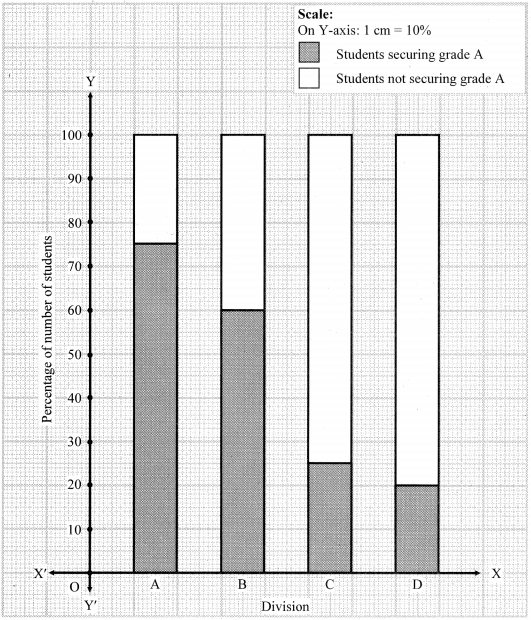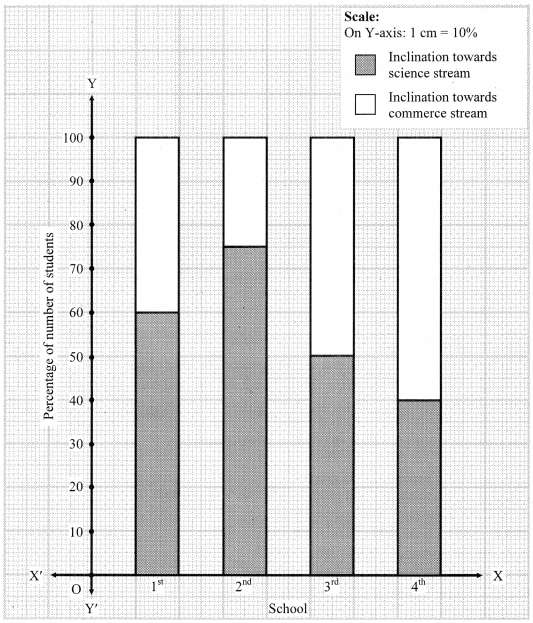# Maharashtra Board Class 8 Maths Solutions Chapter 11 Statistics Practice Set 11.3

## Maharashtra State Board Class 8 Maths Solutions Chapter 11 Statistics Practice Set 11.3

Exercise 11.3 Class 8 Question 1.
Show the following information by a percentage bar graph.

 Division of standard 8 A B C D Number of students securing grade A 45 33 10 15 Total number of students 60 55 40 75

Solution:

 Division of standard 8 A B C D Number of students securing grade A 45 33 10 15 Total number of students 60 55 40 75 Percentage of students securing grade A 75% 60% 25% 20% Percentage of students not securing grade A 25% 40% 75% 80%Statistics for Class 8 Question 2.
Observe the following graph and answer the questions.1. State the type of the bar graph.
2. How much percent is the Tur production to total production in Ajita’s farm?
3. Compare the production of Gram in the farms of Yash and Ravi and state whose percentage of production is more and by how much?
4. Whose percentage production of Tur is the least?
5. State production percentages of Tur and Gram in Sudha’s farm.

Solution:

1. The given graph is a percentage bar graph.
2. Percent of tur production to the total production in Ajita’s farm is 60%.
3. Production of Gram in the farm of Yash = 50%
Production of Gram in the farm of Ravi = 30%
∴ Difference in the production = 50% – 30% =20%
∴ Yash’s production of Gram is more and by 20%.
4. Sudha’s percentage production of Tur is the least.
5. Production percentages of Tur and Gram in Sudha’s farm are 40% and 60% respectively.

8th Standard Statistics Question 3.
The following data is collected in a survey of some students of 10th standard from some schools. Draw the percentage bar graph of the data.

 School 1st 2nd 3rd 4th Inclination towards science stream 90 60 25 16 Inclination towards commerce stream 60 20 25 24

Solution:

 School 1st 2nd 3rd 4th Inclination towards science stream 90 60 25 16 Inclination towards commerce stream 60 20 25 24 Total number of students 150 80 50 40 Percentage of students having inclination towards science stream 60% 75% 50% 40% Percentage of students having inclination towards commerce stream 40% 25% 50% 60%Maharashtra Board Class 8 Maths Chapter 11 Statistics Practice Set 11.3 Intext Questions and Activities

Statistics 8th Class Question 1.
Compare and discuss a percentage bar diagram and a subdivided bar diagram. Use it to learn the graphs in the subjects like Science, Geography. (Textbook pg, no. 74)
Solution:
[Students should attempt the above activity on their own.]